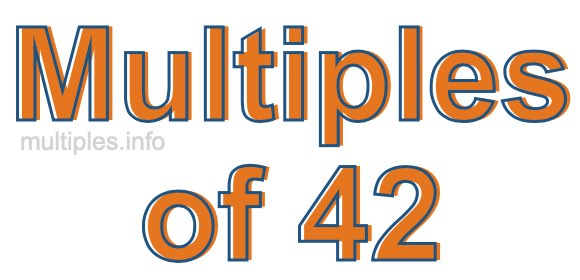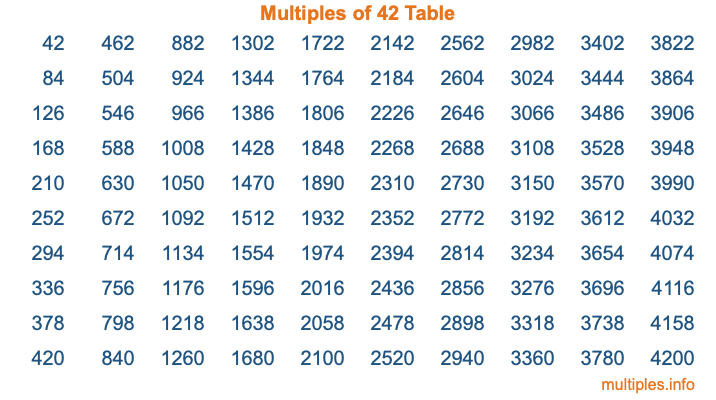Multiples of 42Welcome to the Multiples of 42 page. Here we will first teach you everything you will ever need to know about the multiples of 42, and then give you a study guide summary of everything we taught you to make sure you remember it all. Use this page to look up facts and learn information about the multiples of 42. This page will make you a multiples of forty-two expert!

Definition of Multiples of 42
Multiples of 42 are all the numbers that when divided by 42 equal an integer. Each of the multiples of 42 are called a multiple. A multiple of 42 is created by multiplying 42 by an integer.

Therefore, to create a list of multiples of 42, you start with 1 multiplied by 42, then 2 multiplied by 42, then 3 multiplied by 42, and so on for as long as you want. Thus, the list of the first five multiples of 42 is 42, 84, 126, 168, and 210. To see a larger list of multiples of 42, see the printable image of Multiples of 42 further down on this page. We also have a category where you can choose any nth multiple of 42.

Multiples of 42 Checker
The Multiples of 42 Checker below checks to see if any number of your choice is a multiple of 42. In other words, it checks to see if there is any number (integer) that when multiplied by 42 will equal your number. To do that, we divide your number by 42. If the the quotient is an integer, then your number is a multiple of 42.

Is  a multiple of 42?

Least Common Multiple of 42 and ...
A Least Common Multiple (LCM) is the lowest multiple that two or more numbers have in common. This is also called the smallest common multiple or lowest common multiple and is useful to know when you are adding our subtracting fractions. Enter one or more numbers below (42 is already entered) to find the LCM.

Check out our LCM Calculator if you need more details about the Least Common Multiple or if you need the LCM for different numbers for adding and subtraction fractions.

nth Multiple of 42
As we stated above, 42 is the first multiple of 42, 84 is the second multiple of 42, 126 is the third multiple of 42, and so on. Enter a number below to find the nth multiple of 42.

th multiple of 42

Multiples of 42 vs Factors of 42
42 is a multiple of 42 and a factor of 42, but that is where the similarities end. All postive multiples of 42 are 42 or greater than 42. All positive factors of 42 are 42 or less than 42.

Below is the beginning list of multiples of 42 and the factors of 42 so you can compare:

Multiples of 42: 42, 84, 126, 168, 210, etc.

Factors of 42: 1, 2, 3, 6, 7, 14, 21, 42

As you can see, the multiples of 42 are all the numbers that you can divide by 42 to get a whole number. The factors of 42, on the other hand, are all the whole numbers that you can multiply by another whole number to get 42.

It's also interesting to note that if a number (x) is a factor of 42, then 42 will also be a multiple of that number (x).

Multiples of 42 vs Divisors of 42
The divisors of 42 are all the integers that 42 can be divided by evenly. Below is a list of the divisors of 42.

Divisors of 42: 1, 2, 3, 6, 7, 14, 21, 42

The interesting thing to note here is that if you take any multiple of 42 and divide it by a divisor of 42, you will see that the quotient is an integer.

Multiples of 42 Table
Below is an image of the first 100 multiples of 42 in a table. The table is in chronological order, column by column. The first column has the first ten multiples of 42, the second column has the next ten multiples of 42, and so on.The Multiples of 42 Table is also referred to as the 42 Times Table or Times Table of 42. You are welcome to print out our table for your studies.

Negative Multiples of 42
Although not often discussed or needed in math, it is worth mentioning that you can make a list of negative multiples of 42 by multiplying 42 by -1, then by -2, then by -3, and so on, to get the following list of negative multiples of 42:

-42, -84, -126, -168, -210, etc.

Multiples of 42 Summary
Below is a summary of important Multiples of 42 facts that we have discussed on this page. To retain the knowledge on this page, we recommend that you read through the summary and explain to yourself or a study partner why they hold true.

There are an infinite number of multiples of 42.

A multiple of 42 divided by 42 will equal a whole number.

42 divided by a factor of 42 equals a divisor of 42.

The nth multiple of 42 is n times 42.

The largest factor of 42 is equal to the first positive multiple of 42.

42 is a multiple of every factor of 42.

42 is a multiple of 42.

A multiple of 42 divided by a divisor of 42 equals an integer.

42 divided by a divisor of 42 equals a factor of 42.

Any integer times 42 will equal a multiple of 42.

Multiples of a Number
Here you can get the multiples of another number, all with the same attention to detail as we did for multiples of 42 on this page.

Multiples of
Multiples of 43
Did you find our page about multiples of forty-two educational? Do you want more knowledge? Check out the multiples of the next number on our list!